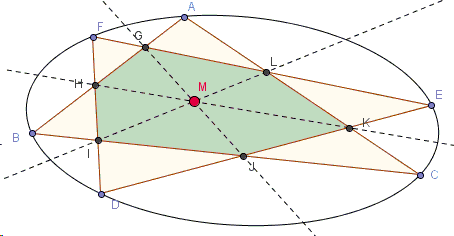# Two Triangles Inscribed in a Conic

Let $ABC$ and $DEF$ be two triangle inscribed in the same conic. Their sides intersect in six points $G, H, I, J, K, L$ as in the diagram.Prove that the lines $GJ$, $HK$, and $IL$ are concurrent.

The applet below serves a dynamic illustration. (The five points that define the ellipse can be displayed and then dragged by checking the "Show 5 pins" box. In case not all intersections of the sides of the triangles are clearly identified, check the box "Show lines".)

### Proof

Let $ABC$ and $DEF$ be two triangle inscribed in the same conic. Their sides intersect in six points $G, H, I, J, K, L$ as in the diagram.Prove that the lines $GJ$, $HK$, and $IL$ are concurrent.

The three lines in question are Pascal's lines of three hexagons obtained from $ABCDEF$ by permutation of indices.

Indeed, $H=AB\cap EF$ while $K=AC\cap DE$ such that $HK$ is the Pascal line of the hexagon $ABEDFC$. (The intersection of $AD$ and $BE$ would be the third point on that line. But we do not need it here.)

Lines $AC$ and $DF$ intersect in $L$, while $BC$ and $DF$ intersect in $I$, making $IL$ the Pascal line of hexagon $ACBEFD$.

Finally, $G=AB\cap EF$ and $J=BC\cap DE$, meaning that $GJ$ is the Pascal line of hexagon $ABCFED$.

However, the proposition does not seem to fall under either Steiner's or Kirkman's theorems covering concurrence of triples of Pascal Lines of an inscribed hexagon $AFBDCE$. For now it's a mystery that might have excited Pascal himself.### Pascal and Brianchon Theorems